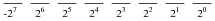# Binary Math

## Digital Circuits

• #### Question 1

Counting practice: count from zero to thirty-one in binary, octal, and hexadecimal:• #### Question 2• #### Question 3

If the numbers sixteen and nine are added in binary form, will the answer be any different than if the same quantities are added in decimal form? Explain.

• #### Question 4

What is the one’s complement of a binary number? If you had to describe this principle to someone who just learned what binary numbers are, what would you say?

Determine the one’s complement for the following binary numbers:

100010102
110101112
111100112
111111112
111112
000000002
000002
• #### Question 5

Determine the two’s complement of the binary number 011001012. Explain how you did the conversion, step by step.

Next, determine the two’s complement representation of the quantity five for a digital system where all numbers are represented by four bits, and also for a digital system where all numbers are represented by eight bits (one byte). Identify the difference that “word length” (the number of bits allocated to represent quantities in a particular digital system) makes in determining the two’s complement of any number.

• #### Question 6

In a computer system that represents all integer quantities using two’s complement form, the most significant bit has a negative place-weight. For an eight-bit system, the place weights are as follows:Given this place-weighting, convert the following eight-bit two’s complement binary numbers into decimal form:

010001012 =
011100002 =
110000012 =
100101112 =
010101012 =
101010102 =
011001012 =
• #### Question 7

In an eight-bit digital system, where all numbers are represented in two’s complement form, what is the largest (most positive) quantity that may be represented with those eight bits? What is the smallest (most negative) quantity that may be represented? Express your answers in both binary (two’s complement) and decimal form.

• #### Question 8

Two’s complement notation really shows its value in binary addition, where positive and negative quantities may be handled with equal ease. Add the following byte-long (8 bit) two’s complement numbers together, and then convert all binary quantities into decimal form to verify the accuracy of the addition:• #### Question 9

Add the following eight-bit two’s complement numbers together, and then convert all binary quantities into decimal form to verify the accuracy of the addition:• #### Question 10

How is it possible to tell that overflow has occurred in the addition of binary numbers, without converting the binary sums to decimal form and having a human being verify the answers?